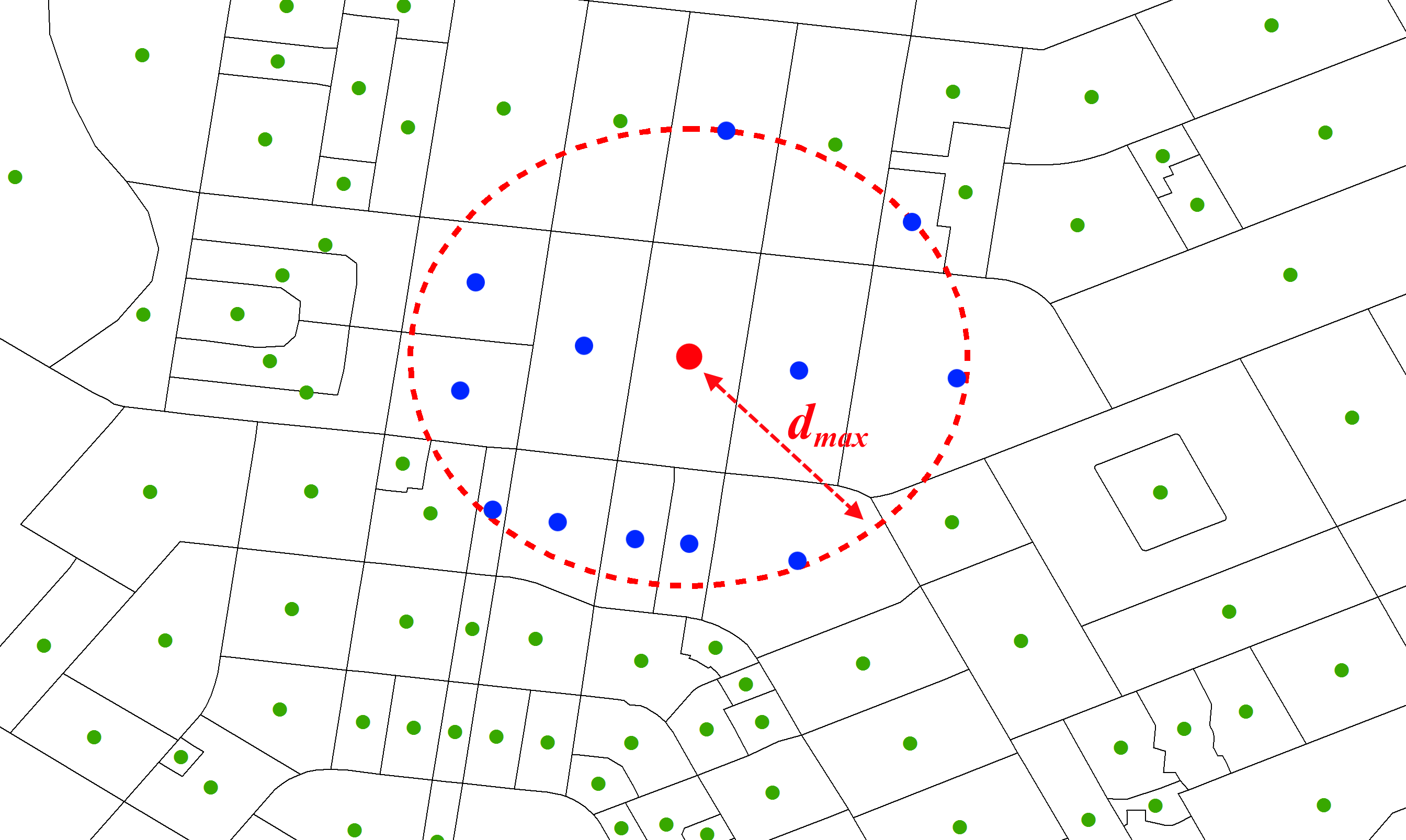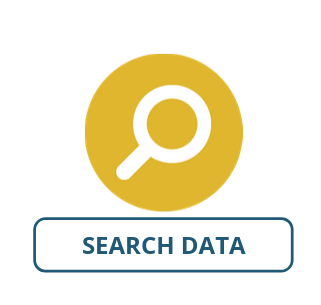Distance Spatial Weight Matrix

There are a number of methods that have been developed to determine the closeness or nearness between locations.

Among some of the easiest to calculate are the “distance” or “threshold” spatial weights matrices. These methods are based on neighbouring areas meeting a specific spatial distance criterion being counted equally as “close”, while all those not meeting the criterion are “not close”. Similar to contiguous spatial weights matrices, all “close” areas are equally weighted, irrespective of their specific distances.

There are two main kinds of Distance Spatial Weights Matrices implemented in AURIN:

K-nearest neighbours

Here we specify that an area is close if it is one of the nearest $k$ number of neighbours to the areas of interest. Normally, for polygons, this is the distance between the centroid of the area of interest and the centroids of the surrounding areas. For the example Canberra mesh blocks below, we have specified that we want to consider the 7 nearest neighbours to be “close” (blue) and all other areas to be “not close” (green).Threshold distance

In contrast to the k-nearest neighbours method, the threshold distance specifies that an area is close if the distance between it and the area of interest $d_{i,j}$ is less than a specified maximum distance, $d_{max}$. If $d_{i,j} > d_{max}$, then the area is not counted as “close”. This is represented by the equation below and illustrated in the figure below, where all areas that are closer to the area of interest (red point) than $d_{max}$ are shown in blue.$w_{i, j} = \left\{\begin{array}{ll} 1 & 0 \leq d_{i,j} \leq d_{max}\\ 0 & d_{i,j} > d_{max} \end{array} \right.$

SET UP

To illustrate the Distance Spatial Weights Matrix in use, we will use socio-economic data from the Australia Bureau of Statistics for Perth.

• Select Greater Perth GCCSA as your area
• Select ABS – Socio-Economic Indexes for Areas (SEIFA) – The Index of Relative Socio-Economic Advantage and Disadvantage (SA2) 2011 as your dataset, selecting all of the variables.

Spatialise the above dataset using the Spatialise Aggregated Dataset tool, or the Spatialise Dataset tool in the spanner icon next to the dataset.

Inputs

After spatialising the dataset, open the Distance spatial weights matrix tool (Tools → Spatial Weight Matrices → Distance) and enter the parameters as listed below:

• Dataset Input: The dataset that you would like to calculate the spatial weights matrix on. This must be a dataset with included geometries, either created from spatialising the dataset as above, or uploading a shapefile. Select the spatialised dataset generated above.
• Key Column: The unique identifier for each row. Select SA2 Code.
• Type: This specifies whether you want k-nearest neighbours or threshold distance, as described above. Select distance-threshold.
• Threshold Distance or k Parameter: This specifies how many neighbours, or the threshold distance (in kilometers) for your matrix, depending on which of the matrix types you choose. Type 50.
• Style: This specifies whether the standardisation will be binary, row-standardised, or globally-standardised. Select row-standardised.
• Island Parameter: This specifies how the matrix will deal with islands, which naturally have no contiguous neighbours. Tick the box.Once you have entered your parameters, click Run Tool.

Outputs

Once you have executed the tool, you should see an output appear in your Data panel. If you open this, you should see something like this:“0” scores within a cell indicate that the areas are not classified as “close”, and therefore do not affect each other. If a value is greater than zero, it indicates that the area specified by the column influences the area of the row by that much. It is important to realise that the values along the row will be identical – that is, each area that is “near to” the target area influences it equally.

This spatial weights matrix can now be used for additional spatial statistical analysis, such as Moran’s I or Geary’s C.

Looking for Spatial Data?

You can browse the AURIN Data Discovery:How can you Create Impact?